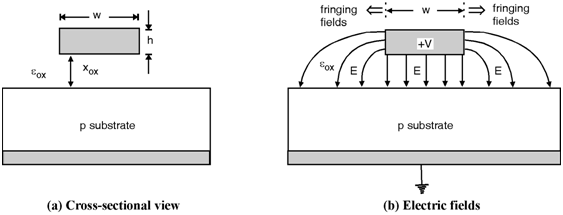Home > System On Chip > Interconnects > Wire Capacitance

Wire Capacitance

The interconnect capacitance is the limiting factor in high-speed circuits. Consider the circuit shown in figure below. The capacitance equations used here are having unit capacitance per unit length i.e farads per centimetre. The total wire capacitance is given by,

Cwire = C×dFig_Wire Capacitance

The expression for C is given by as,

C = 0x wXox

The unit of c in above equation is F/cm. However, since this formula ignores the fringing electric fields at the edges. The modified equation of C is given by,

C = ox 1.15 wXox + 2.8 hXox0.222 F/cm.

Therefore, the value of capacitance is higher by including the fringing fields shown in figure.